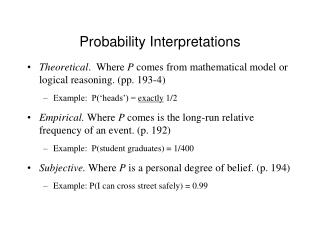DownloadDownload PresentationProbability Interpretations

# Probability Interpretations

Download Presentation## Probability Interpretations

- - - - - - - - - - - - - - - - - - - - - - - - - - - E N D - - - - - - - - - - - - - - - - - - - - - - - - - - -
##### Presentation Transcript

1. Probability Interpretations • Theoretical. Where P comes from mathematical model or logical reasoning. (pp. 193-4) • Example: P(‘heads’) = exactly 1/2 • Empirical. Where P comes is the long-run relative frequency of an event. (p. 192) • Example: P(student graduates) = 1/400 • Subjective. Where P is a personal degree of belief. (p. 194) • Example: P(I can cross street safely) = 0.99

2. Complement Rule • The probability that an event occurs is l minus the probability that it doesn't occur. (p. 195) P(A) = 1 – P(~A) or P(A) + P(~A) = 1

3. Example: Complement Rule P(heads) = 1 – P(tails) P(heads) + P(tails) = 1

4. General Addition Rule For any two events Aand B, the probability of A or B occurring is: P(A or B) = P(A) + P(B) – P(A and B).

5. Example: General Addition Rule Flip a coin twice. Probability of 2 heads: P(heads, heads) = 0.5 + 0.5 – 0.25 = 0.75.

6. Mutually Exclusive Events • If Aand B are mutually exclusive (disjoint), then if Aoccurs, B cannot occur. (p. 196)

7. Example: Mutually Exclusive Events • Being registered as Republican vs. Democrat vs. Independent are mutually exclusive events. • Freshman, Sophomore, Junior, Senior • CA driver’s license lists gender as M or F • Rolling a ‘1’, ‘2’, ‘3’, ‘4’, ‘5’, or ‘6’ on fair die

8. Addition Rule for Mutually Exclusive Events If Aand B are mutually exclusive events, then the probability of A or B is: P(A or B) = P(A) + P(B). p. 196

9. Example: Addition Rule for Mutually Exclusive Events Probability of rolling a ‘1’ or a ‘2’ on a fair die: 1/6 + 1/6 = 1/3

10. General Multiplication Rule • For any two events (A, B), the probability of A and B is: P(A and B) = P(A) × P(B|A) p. 202

11. Example: General Multiplication Rule • Probability of being a Democrat and likes president: = P(Democrat) × P(likes president|Democrat) = 0.50 × 0.80 = 0.40

12. Independent Events • Events are independent if one event’s occurring doesn’t change the probability of the other’s occurring. If A and B are independent, then: P(B|A) = P(B|~A) = P(B) p. 202

13. Example: Independent Events • Getting ‘heads’ on one coin clip independent of getting ‘heads’ on another. • Rolling a ‘1’ on a fair die independent of getting ‘heads’ on a coin clip. P(‘1’|heads) = P(‘1’|tails) = P(‘1’)

14. Multiplication Rule for Independent Events • If A and B are independent, the probability of A and B is: P(A and B) = P(A) × P(B) p. 202

15. Example: Multiplication Rule for Independent Events • Probability of getting ‘heads’ on both 1st and 2nd of two coin flips: P(heads, heads) = P(heads) × P(heads) = 0.5 × 0.5 = 0.25

16. Conditional Probability • The conditional probability P(B|A) is the probability of B given that A occurs. P(A and B) P(B|A)= P(A) pp. 202-203

17. Example: Conditional Probability Table 1. Purchase Type by Gender P(male customer|buys utility lighting) = 0.40/0.50 = 0.80.